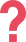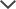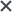If you have any questions, feel free to contact Eitan — TestPrep-Online's CAT4 expert — at ask_eitan@testprep-online.com

## About the CAT 4 Level D

The Cognitive Abilities Test fourth edition (CAT4) is designed to help schools understand their students’ abilities and how to develop them. The test helps identify individual students' strengths and weaknesses while also monitoring the performances of different groups of students. In addition, the CAT4 is widely used as an entrance exam to many luxurious schools in the UK and Ireland as well as to international schools around the world. The CAT4 exam is administered to students from age 6 to 17, and the Level D version is designated for year 7 in the UK, catering to children between the ages of 10 to 12.

It is comprised of the following sections:

• Quantitative Reasoning-Number Series and Number Analogies
• Verbal Reasoning-Verbal Classification and Verbal Analogies
• Non-Verbal Reasoning-Figure Classification and Figure Matrices
• Spatial Ability-Figure Analysis and Figure Recognition

## CAT 4 Level D Sample Questions

 CAT4 Level D Test Practice Question-Quantitative Reasoning #1 [15 → 50]        [10 → 35]        [7 → ?] 21 22 25 26 32 Answer & Explanation ▼ | ▲ The correct answer is 26. The simple possible connection between the numbers in the first pair (15 → 50) is: 15 + 35 = 50 However, the second pair (10 → 35) does not share that same connection: 10 + 35 ≠ 35 (notice that the difference is 25: 10 + 25 = 35) Therefore, there must be a more complex connection. Since 50 is not divisible by 15, a possible connection could be using multiplication and addition/subtraction. Some of the possibilities are: 15 × 2 + 20 = 50 15 × 3 + 5 = 50 15 × 4 – 10 = 50 More connections can be found; however, you should try the simplest ones first. Checking those connections on the second pair shows: 10 × 2 + 20 = 40 ≠ 35 10 × 3 + 5 = 35 10 × 4 – 10 = 30 ≠ 35 The rule which applies for both pairs is the second one, which is: X × 3 + 5, where X is the number on the left side of the pair. Using this rule on the third pair, you can find the answer: 7 × 3 + 5 = 26 Therefore, the correct answer is 26. #Note that additional practice questions for the Quantitative Reasoning battery (number analogies + number series) can be found on our CAT4 Level D practice packs.

 CAT4 Level D Test Practice Question-Verbal Reasoning #1 Halt    conclude    cease walk slowdown decelerate go pause Answer & Explanation ▼ | ▲ The correct answer is ‘pause’. All the words above describe the action of stopping in a certain context. The word ‘pause’ means to stop temporarily, so it fits the category. ‘Walk’ and ‘go’ are incorrect because they describe things that move forward. ‘Slowdown’ and ‘decelerate’ are incorrect because they describe something that is decreasing speed, but not actually stopping. #Note that additional practice questions for the Verbal Reasoning battery (Verbal analogies + Verbal classification) can be found on our CAT4 Level D practice packs.

CAT4 Level D Test Practice Question-Non Verbal Reasoning #1In the top row we have two figures - how do they go together?

We can see that the two figures look similar, but:

• On the right, all the shapes are closed and not open,
• The outer shape has received another side,
• The middle shape has become more rectangular, and –
• The inner shape has been flipped on its side.

In the bottom row, we have a single figure, and we need to find a figure that will go together with it in the same way that the two figures above go together.

We can immediately eliminate answer choice 3 because it belongs to the family of figures in the top row, and not the bottom row.

We can also eliminate answer choice 2 because it has the same number of sides as the first shape.

When we look at the other three answer choices, the only one that matches the pattern is answer choice 1.

Answer choice 4 includes open shapes and not closed ones.

Answer choice 5 includes nearly all the changes we need, but the triangle is not flipped on its side.

#Note that additional practice questions for the Non-Verbal Reasoning battery (Figure analogies + Figure classification) can be found on our CAT4 Level D practice packs.

CAT4 Level D Test Practice Question-Spatial ability #1• First, the paper was folded diagonally in half from the upper-right corner to the lower-left corner. Then it was folded again from the upper-left corner to the lower-right corner.
• Then, four holes were cut out of four-layer of paper.
• Therefore, when the paper is unfolded it will have sixteen holes (4 holes x 4 layers) which will mirror each other across the diagonals of the paper like this:Answer choice 4 has fewer than sixteen holes, so it can be eliminated.

Answer choice 1 has more than sixteen holes, so it can be eliminated as well.

Looking at answer choice 2, we can see that although it has the right number of holes, they are in the wrong place. This can be seen when we compare the location of the holes on the bottom of the square to the ones in the original image above.

In answer choice 3, we can see that the holes on the sides of the square are placed in the same position, and do not fit the diagonal mirroring pattern we need.

We are left with the 5th choice, which is the correct answer.

#Note that additional practice questions for the Spatial Ability battery (Paper folding + Figure recognition) can be found on our CAT4 Level D practice packs.## CAT 4 Format & Sections

CAT4 is a multiple-choice test that consists of four test batteries, each containing two short sub-tests:

 Verbal Reasoning Verbal ClassificationVerbal Analogies Quantitative Reasoning Number AnalogiesNumber Series Non-verbal Reasoning Figure ClassificationFigure Matrices Spatial Ability Figure AnalysisFigure Recognition

These short tests are administered in three parts:

 Part 1 Question Number Test Time Figure Classification 24 questions 10 minutes Figure Matrices 24 questions 10 minutes Part 2 Verbal Classification 24 questions 8 minutes Verbal Analogies 24 questions 8 minutes Number Analogies 18 questions 10 minutes Part 3 Number Series 18 questions 8 minutes Figure Analysis 18 questions 9 minutes Figure Recognition 18 questions 9 minutes

## Prepare for the CAT 4 Level D Exam with TestPrep-Online

The CAT4 has a unique question format and strict time limitations, making it seem daunting to many students. Practicing prior to the exam can help you feel more comfortable with the testing format and the various question types. Properly preparing can also give you the advantage of recognizing your strengths and weaknesses, and it can help eliminate the feeling of stress under time constraints.

Practicing with TestPrep Online's CAT4 Level D PrepPack allows you to familiarize yourself with the test and gives you the confidence needed to succeed. The premium pack includes two realistic full-length CAT4 Level D simulations that familiarize you with the test’s unique structure, alongside multiple section-specific additional drills and a comprehensive study guide with solving tips and strategies.

Upon finishing each practice test in the pack, you can review your test using a detailed score report that shows the total number of correct and incorrect answers, detailing which ones were answered correctly and which incorrectly. With this report, you can identify your strengths and weaknesses and determine where to focus your efforts to become fully prepared in all the various topics on the test.

In addition to viewing the score report, you can also read full explanations for each question. For questions that have been answered incorrectly, the explanation can be used to understand what was done wrong and how to correctly solve it. For questions that have been answered correctly, the explanation can still help introduce you to new methods and approaches for solving those types of questions.

The CAT4, CogAT, and other trademarks are the property of their respective trademark holders. None of the trademark holders are affiliated with TestPrep-Online or this website.

Need HelpNeed Help